# Create Heat Map from Comparison

The Create Heat Map from Comparison tool builds a heat map from a Pairwise Comparison such as those generated by Create Average Nucleotide Identity Comparison tool.

To run the Create Heat Map from Comparison tool:

Toolbox | Whole Genome Alignment (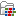) | Create Heat Map from Comparison (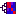)

Once the tool wizard has opened (figure 6.1), choose the Pairwise Comparison table you would like to use.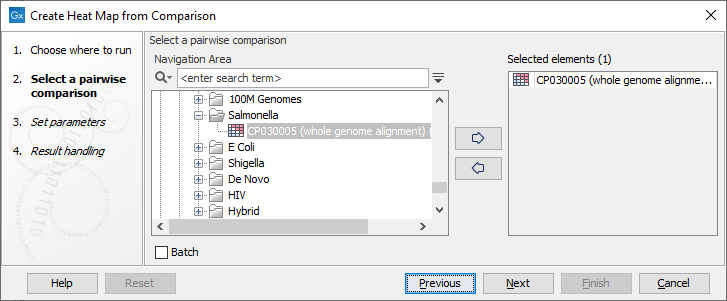Figure 6.16: Select a Pairwise Comparison table.

In the next dialog (figure 6.2), you can set the following parameters: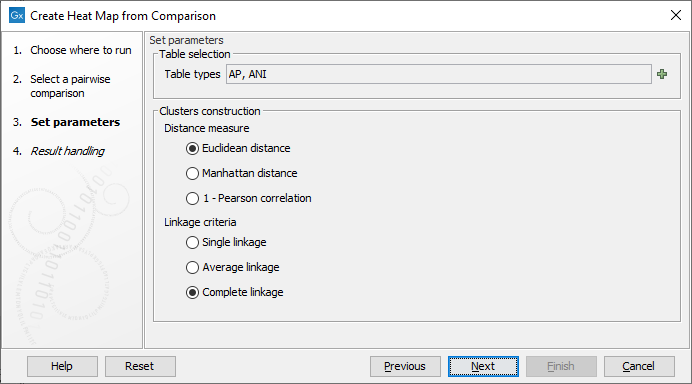Figure 6.17: Select the table types and clusters construction methods you would like to use for building the heat maps.

• Table types The possible table types are extracted from the Pairwise Comparison table input. In the case of a Pairwise Comparison table obtained from Create Average Nucleotide Identity Comparison, these are: ANI (Average Nucleotide Identity) or AP (Alignment Percentage). If left empty, as it is by default, both types will be used.
• Clusters construction methods

There are three kinds of Distance measures:

• Euclidean distance. The ordinary distance between two points - the length of the segment connecting them. If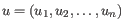and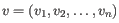, then the Euclidean distance between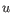and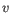is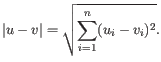• 1 - Pearson correlation. The Pearson correlation coefficient between two elements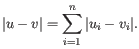and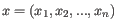is defined as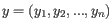where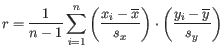is the average of values in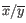and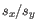is the sample standard deviation of these values. It takes a value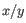. Highly correlated elements have a high absolute value of the Pearson correlation, and elements whose values are un-informative about each other have Pearson correlation 0. Usingas distance measure means that elements that are highly correlated will have a short distance between them, and elements that have low correlation will be more distant from each other.
• Manhattan distance. The Manhattan distance between two points is the distance measured along axes at right angles. Ifand, then the Manhattan distance betweenandis• Single linkage. The distance between two clusters is computed as the distance between the two closest elements in the two clusters.
• Average linkage. The distance between two clusters is computed as the average distance between objects from the first cluster and objects from the second cluster. The averaging is performed over all pairs, whereis an object from the first cluster andis an object from the second cluster.
• Complete linkage. The distance between two clusters is computed as the maximal object-to-object distance, wherecomes from the first cluster, andcomes from the second cluster. In other words, the distance between two clusters is computed as the distance between the two farthest objects in the two clusters.

The Pairwise Comparison table input is either a distance or similarity matrix. The tool automatically detects the type of each table by checking the values on the diagonal: if the diagonal contains only zeros, then the table represents a distance matrix, otherwise a similarity matrix. If the table is distance matrix, a similarity matrix s is calculated as follows:

s[i][j] = min + (1 - t[i][j]) * (max - min) if the table is a distance matrix,

where t[i][j] is the relative value (between 0 and 1) found in the table in row i and column j,

and min and max are the minimum and maximum magnitude of the table.

A heat map (figure 6.3) is then created from the similarity matrix s according to the specified clustering options and using a hierarchical clustering algorithm. Note that the tool outputs a heat map for each chosen table type and its name contains the table type used.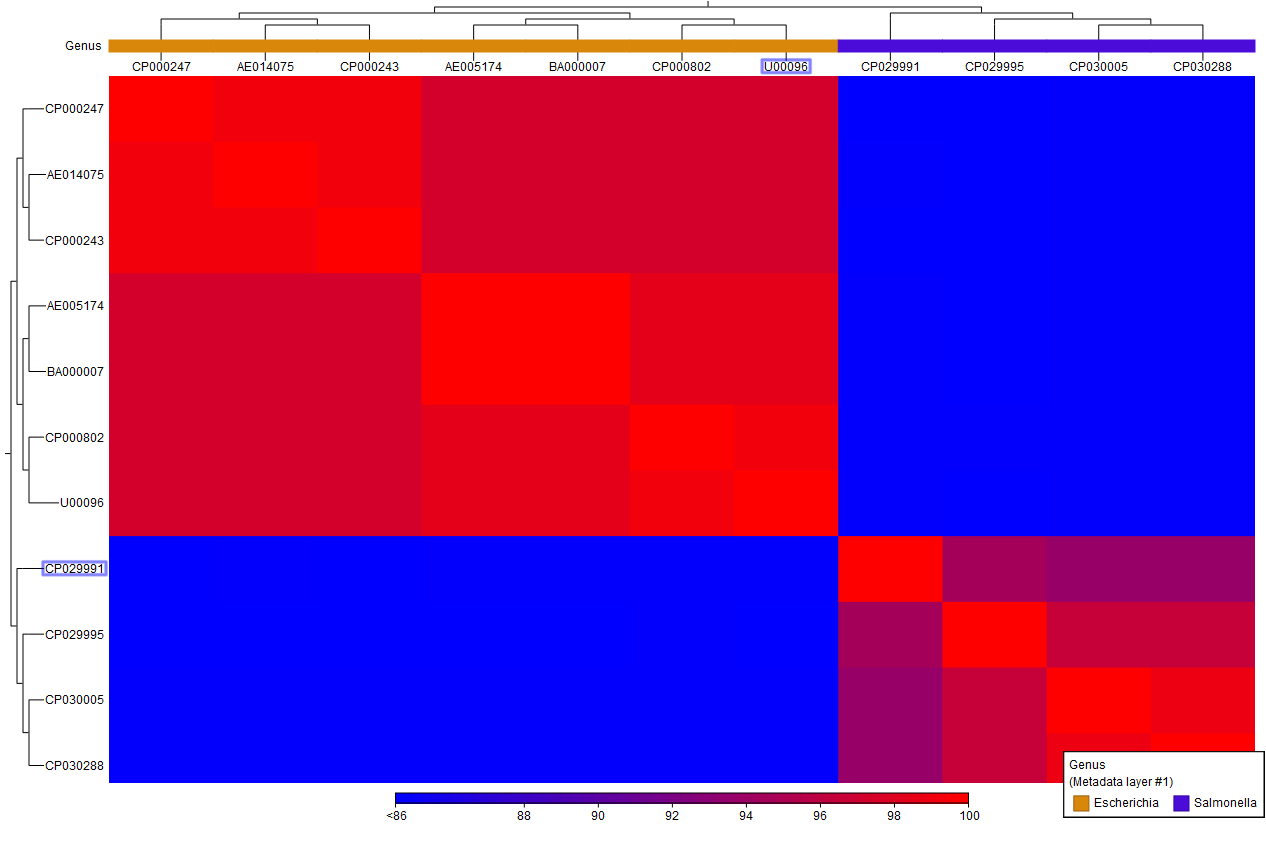Figure 6.18: A Comparison Heat Map.